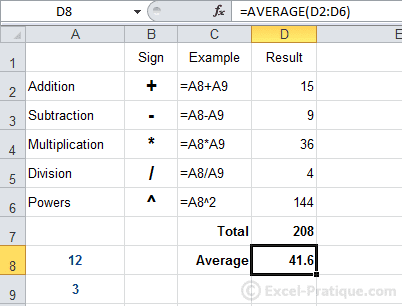# Excel Course: Formula Calculations and Functions

## Syntax for Formula Calculations

Always start your calculation formulas with the `=` sign, then without any spaces, enter a number followed by an operator, followed by another number, etc. Add `( )` if needed.

Examples:

`=45-32` (Excel will display the result: 13)

`=(45-32)^2/10` (Excel will display the result: 16.9)

Take a look at this table: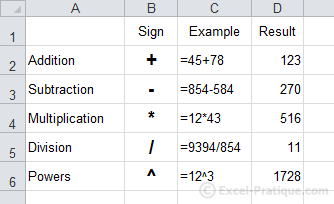Calculations can also be made using data that comes from other cells.

In this case, start with an `=` sign, then click on a first cell, add an operator, click on another cell, etc. Add `( )` if needed.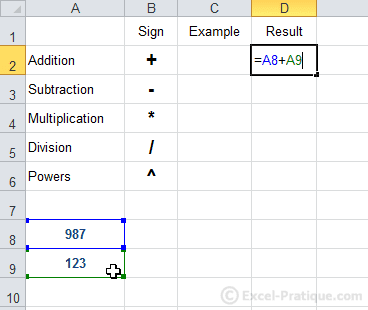Take a close look at these formulas: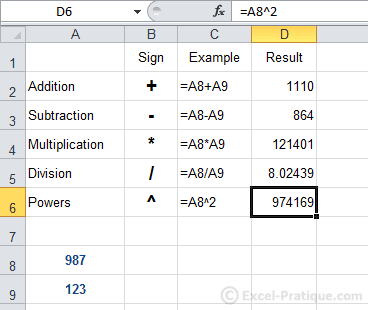The formulas in the table shown below use the values of A8 and A9. The main advantage of this approach is that the results will be updated automatically (without changing the formulas) simply by changing the values in A8 and A9, for example: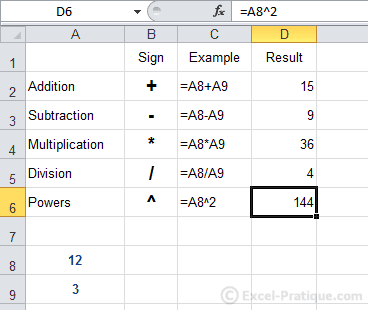## Introduction to Functions

At this point, we will introduce you to just a few functions so that you can see how they work.

There are an incredible number of functions. You will find explanations of the most commonly used functions on the Functions page (you can reach it by clicking on "Functions Explained" at the top of the page).

## SUM Function

Select the cell in which you would like to display the sum. Then click on the "Formulas" tab, and then on "AutoSum".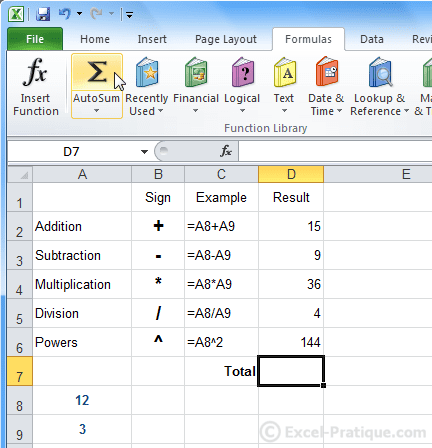Then select the range of cells from which you would like to calculate the sum (in this case, the range suggested by Excel is correct).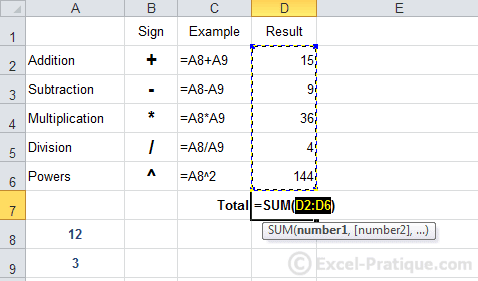The sum of cells D2 to D6 has already been calculated.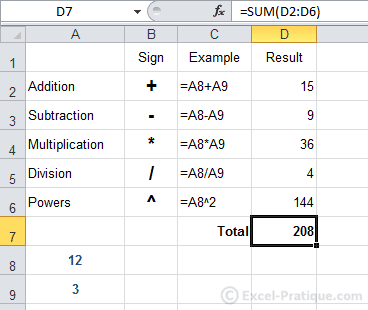## AVERAGE Function

There are all kinds of shortcuts for inserting functions in the "Formulas" tab. This time, we'll insert a function without using the shortcuts at all ...

Select the cell where you would like to display the avergage and click on "Insert a function":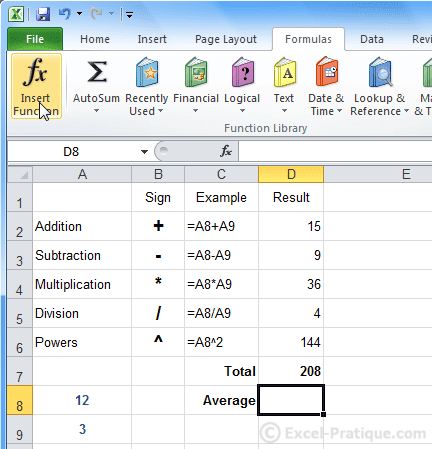Find and select the AVERAGE function: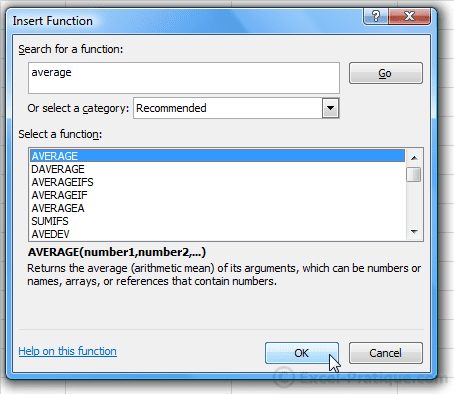Click OK. The function window will appear.

Select the range of cells from which to calculate the average.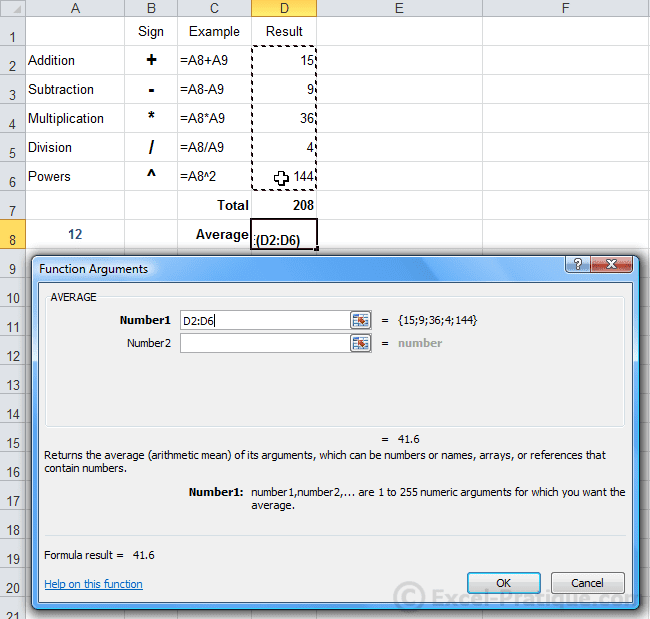You will see this result: